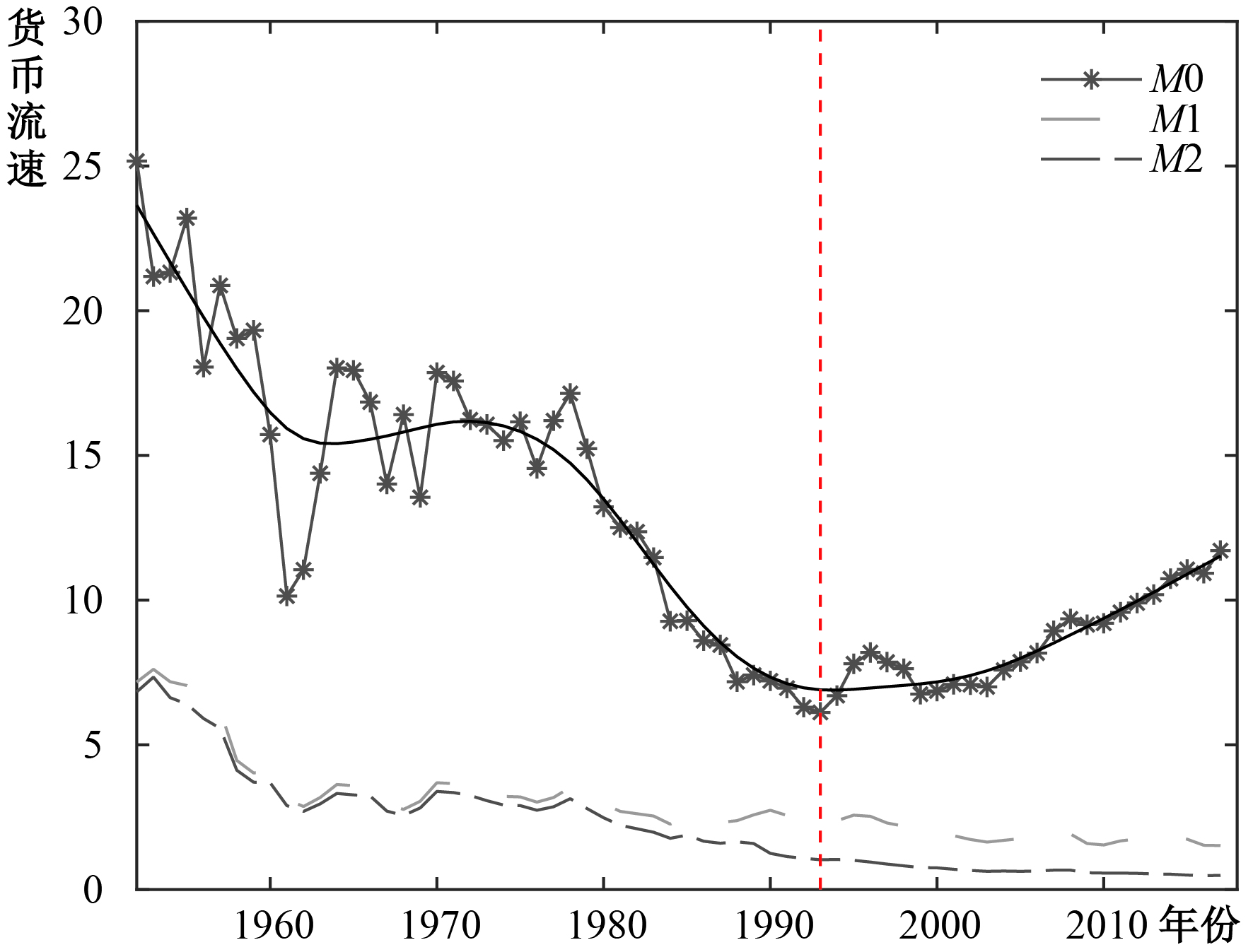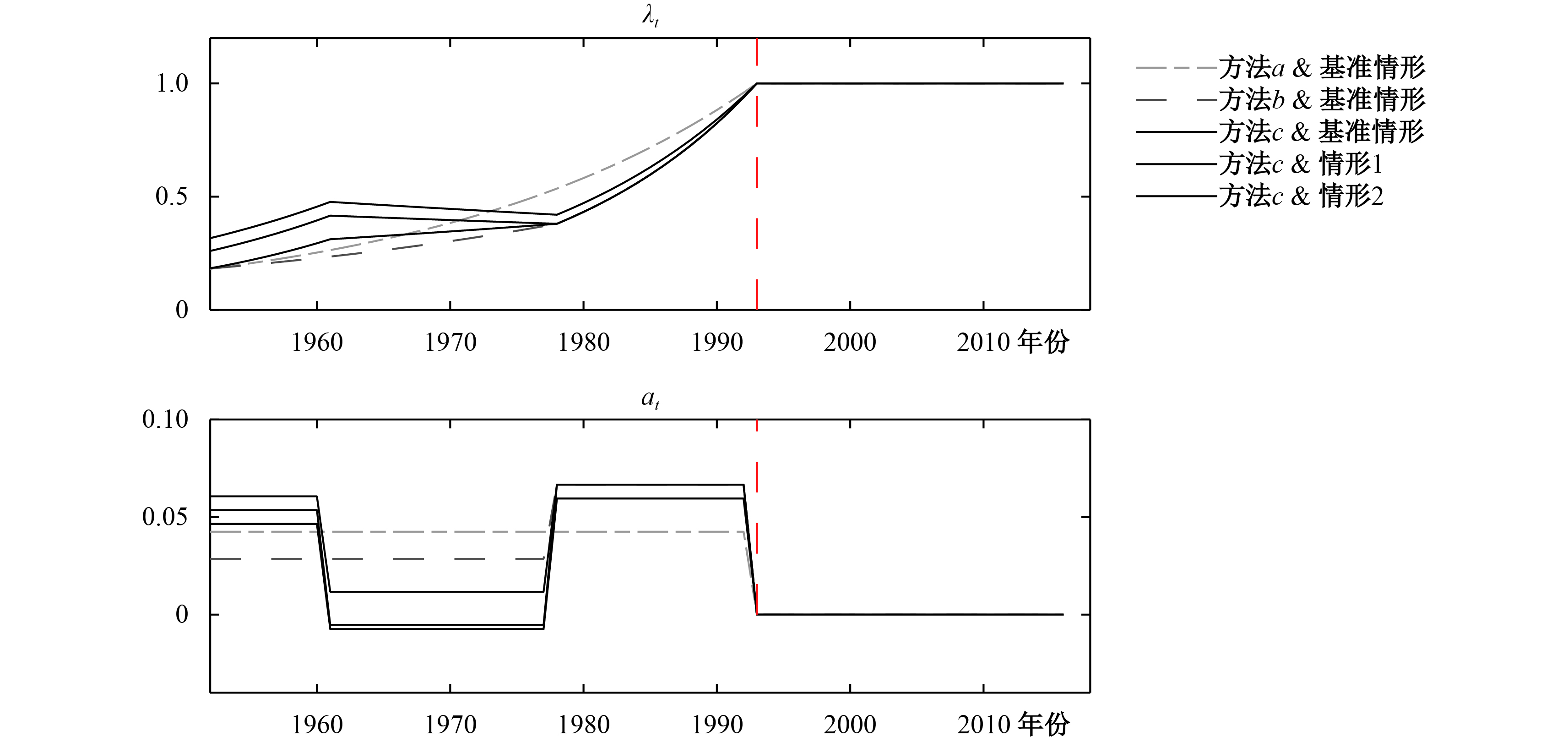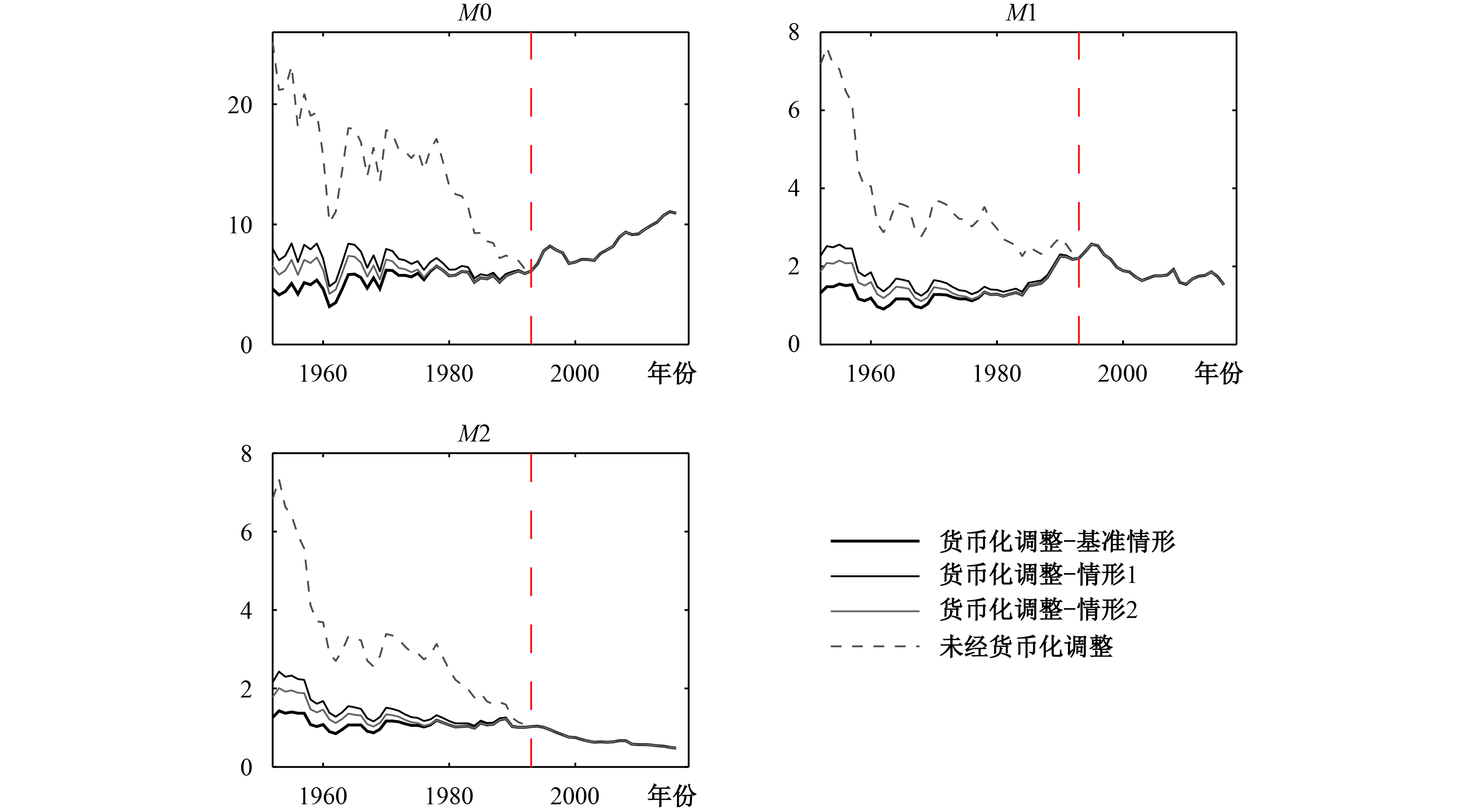﻿ <b>货币化率的直接测算及土地与住房的货币化</b>——中国经济改革中的货币化进程再思考
 财经研究2018, Vol. 44Issue (6): 31-490

#### 文章信息财经研究2018年44卷第6期

Liu Jianfeng, Xu Zhiwei, Pan Yingli.

The direct calculation of monetization rate & the monetization of land and housing: a reconsideration of the monetization process in china during the economic reform

Journal of Finance and Economics, 2018, 44(6): 31-49.

### 文章历史《财经研究》
2018第44卷第6期

The Direct Calculation of Monetization Rate & the Monetization of Land and Housing: A Reconsideration of the Monetization Process in China during the Economic Reform
Liu Jianfeng, Xu Zhiwei, Pan Yingli
Antai College of Economics & Management，Shanghai Jiao Tong University，Shanghai 200030，China
Key words: proportion of monetized economy    velocity of money    money missing puzzle    the excessive monetization of land & housing    underground economy图 1 1952−2016年传统定义的各层次货币流速的变化趋势 注：1952−1999年各层次货币供应量数据来自历年的《中国金融年鉴》，2000年之后的数据来自中国人民银行官网。名义GDP数据来自Chang等（2016）。图中实线为经过HP滤波（平滑参数为100）过滤后的传统M0流速的长期趋势，虚竖线对应1993年。

 国家 2011年 2012年 2013年 2014年 2015年 柬埔寨 39.07 50.11 52.88 62.96 66.87 缅甸 26.41 31.67 36.77 39.54 46.33 印度尼西亚 36.74 38.39 39.08 39.48 39.45 印度 81.66 82.88 83.64 84.09 − 巴基斯坦 37.49 40.00 41.11 40.59 − 阿富汗 36.37 31.93 32.71 34.90 34.43 伊朗 52.10 46.49 47.00 56.81 − 埃及 75.79 70.48 75.28 76.45 78.44 苏丹 23.52 26.23 21.01 18.35 − 南非 59.50 57.44 57.90 58.48 60.28 中非 19.23 18.26 28.12 28.74 − 越南 99.80 106.46 117.03 127.55 137.65 菲律宾 77.53 79.42 99.16 103.15 106.23 马来西亚 133.33 137.02 141.02 139.56 137.28 泰国 119.93 121.12 124.31 127.31 128.37 注：印度的统计口径为M3/GDP。数据来源：Wind资讯。

1952—2016年的宏观数据（见图1）显示，传统定义的M1和M2流速呈现单调下降趋势，而M0流速则在1993年出现明显的V形转折点，1993年之前呈现长期下降趋势，之后则逐渐上升。我们观察发现，M0流速这种特有的时间序列特征包含了与货币化进程相关的重要信息，而这一点往往被以往的文献所忽视。图 2 2008年经济危机后房地产行业新增人民币贷款占比与产出占比 数据来源：Wind资讯。

（一）Yi（1991）包含货币化率的货币数量方程及其扩展

 $V_{it} = {{GD{P_{obt}}} / {M{i_t}}},{\text{ }}i \in \left\{ {0,1,2} \right\}$ (1)

${\lambda _t}$ 为我国在第t年的货币化率，定义包含不可观测地下经济活动的广义名义GDPt $GD{P_t} = {P_t}{Y_t}$ ，其中 ${Y_t}$ 为广义真实GDP）与货币化率的乘积（ ${\lambda _t}GD{P_t}$ ）为已货币化的广义名义GDP。根据Yi（1991）的研究，考虑货币化因素后，可定义各层次货币的真实流速（记为 ${\tilde V_{it}}$ ）为已货币化的广义名义GDP除以各层次货币供应量（ $M{i_t}$ ）的数值：

 ${\tilde V_{it}} = {{({\lambda _t}GD{P_t})} / {M{i_t}}},{\text{ }}i \in \left\{ {0,1,2} \right\}$ (2)

 $GD{P_t} = {e^{{\phi _{unobt}}}}GD{P_{obt}}$ (3)

 ${V_{it}} = {{{e^{ - {\phi _{unobt}}}}{{\tilde V}_{it}}} / {{\lambda _t}}},{\text{ }}i \in \left\{ {0,1,2} \right\}$ (4)

（二）货币化进程完成的两个基本定义

$t \in [{t_l},{t_u}]$ ，我们从货币化因素的水平值和变化值角度给出“货币化进程完成”的两个基本定义。

 {V_{0t}}=\left\{ {\begin{aligned}& {{{{\dot \varepsilon }_t}} /{{\lambda _t}}},\quad{\text{for }}[t,T),t {\text{≥}} {t_l},{\lambda _t} \in \left( {0,1} \right) \\& {{\dot \varepsilon }_t},\quad\quad\; {\text{for }}[T,t],t {\text{≤}} {t_u},{\lambda _t} \equiv 1 \\ \end{aligned}} \right. (5)

 {\tilde V_{0t}} = {\dot \varepsilon _t}{e^{{\phi _{unobt}}}},{V_{0t}}={{GD{P_{obt}}} / {M{0_t}}} = \left\{ {\begin{aligned}& {{{e^{ - {\phi _{unobt}}}}{{\tilde V}_{0t}}} / {{\lambda _t}}},{\text{ for }}[t,T),t {\text{≥}} {t_l},{\lambda _t} \in \left( {0,1} \right) \\& {e^{ - {\phi _{unobt}}}}{{\tilde V}_{0t}},\;\;\;\;\; {\text{ for }}[T,t],t {\text{≤}} {t_u},{\lambda _t} \equiv 1 \end{aligned}} \right. (6)

 E({a_t}) = \left\{ \begin{aligned}& {a_{tT}} > 0, \;{\rm{for }}\;[t,T),t {\text{≥}} {t_l},{\lambda _t} \in \left( {0,1} \right)\\& 0, \;\;\;\;\;\;\;\;\;\;\,{\rm{for }}\;[T,t],t {\text{≤}} {t_u}, {\lambda _t} \equiv 1\end{aligned} \right. (7)

（三）三个基本假设

 ${\dot \phi _{unobt}}\sim (0,\sigma _{\phi t}^2),{\text{ }}E({\dot \phi _{unobt}}) = 0$ (8)

 ${{{{\ddot \varepsilon }_t}} / {{{\dot \varepsilon }_t}}}\sim ({\mu _{ij}},\sigma _{\varepsilon ij}^2),{\text{ }}E({{{{\ddot \varepsilon }_t}}/ {{{\dot \varepsilon }_t}}}) = {\mu _{ij}} > 0,{\text{ }}t \in [{t_i},{t_j}]$ (9)

 ${{{{\dot {\tilde V}}_{0t}}} / {{{\tilde V}_{0t}}}} - {\dot \phi _{unobt}}\sim ({\mu _{ij}},\sigma _{\varepsilon ij}^2),{\text{ }}E({{{{\dot {\tilde V}}_{0t}}} / {{{\tilde V}_{0t}}}}) = {\mu _{ij}} > 0,{\text{ }}t \in [{t_i},{t_j}]$ (10)

 $E({a_t}) > E({{{{\ddot \varepsilon }_t}} / {{{\dot \varepsilon }_t}}}),{\text{ for }}[t,T),t {\text{≥}} {t_l},{\lambda _t} \in \left( {0,1} \right)$ (11)

（四）命题 $T = {T_1}$ 的证明

 E({{{{\dot V}_{0t}}} / {{V_{0t}}}}{\text{) = }}\left\{ {\begin{aligned}& E({{{{\ddot \varepsilon }_t}} / {{{\dot \varepsilon }_t}}}) - E({{{{\dot \lambda }_t}} / {{\lambda _t}}}), {\text{ for }}[t,T),t {\text{≥}} {t_l},{\lambda _t} \in \left( {0,1} \right) \\& E({{{{\ddot \varepsilon }_t}} / {{{\dot \varepsilon }_t}}}), \quad\quad\quad\quad\ {\text{ for }}[T,t],t {\text{≤}} {t_u},{\lambda _t} \equiv 1 \end{aligned}} \right. (12)

 E({{{{\dot V}_{0t}}} / {{V_{0t}}}}{\text{) = }}\left\{ {\begin{aligned}& E({{{{\dot {\tilde V}}_{0t}}} / {{{\tilde V}_{0t}}}}) - {a_{tT}}={\mu _{tT}} - {a_{tT}},{\text{ for }}[t,T),t {\text{≥}} {t_l},{\lambda _t} \in \left( {0,1} \right) \\& E({{{{\dot {\tilde V}}_{0t}}} / {{{\tilde V}_{0t}}}})={\mu _{Tt}},\quad\quad \quad\quad{\text{ for }}[T,t],t {\text{≤}} {t_u},{\lambda _t} \equiv 1\end{aligned}} \right. (13)

 E({{{{\dot V}_{0t}}} / {{V_{0t}}}}{\text{) }}\left\{ {\begin{aligned}& < 0,{\text{ for }}[t,T),t {\text{≥}} {t_l},{\lambda _t} \in \left( {0,1} \right) \\& > 0,{\text{ for }}[T,t],t {\text{≤}} {t_u},{\lambda _t} \equiv 1 \end{aligned}} \right. (14)

 E({\dot V_{0t}}{\text{) }}\left\{ {\begin{aligned}& < 0,{\text{ for }}[t,T),t {\text{≥}} {t_l},{\lambda _t} \in \left( {0,1} \right) \\& > 0,{\text{ for }}[T,t],t {\text{≤}} {t_u},{\lambda _t} \equiv 1 \end{aligned}} \right. (15)

 E({\dot V_{0t}})\left\{ {\begin{aligned}& < 0,{\text{ for }}[t,{T_1}),t {\text{≥}} {t_l} \\& > 0,{\text{ for }}[{T_1},t],t {\text{≤}} {t_u} \\ \end{aligned}} \right. (16)

（一）测算货币化率

1. 测算方法a

 ${{\hat {\lambda}} _t} = 1,{\text{ }}{\hat a_{93}} = \cdots = {\hat a_{16}} = 0, t \in \left[ {1993,2016} \right]$ (17)

 ${\hat \mu _{93 - {\text{15}}}} = \% \hat V_{93 - 15}^0 = {{(\sum\nolimits_{t = 1993}^{2015} {hp({{\Delta {V_{0t}}} / {{V_{0t - 1}}}})} )} /{(2015 - 1993{\text{ + }}1)}} = 2.12\%$ (18)
 $\% \hat V_{52 - 92}^0 = {{(\sum\nolimits_{t = 1952}^{1992} {hp({{\Delta {V_{0t}}} / {{V_{0t - 1}}}})} )} / {(1992 - 1952{\text{ + }}1)}} = - 2.13\%$ (19)

 ${\text{ }}E({{{{\ddot \varepsilon }_t}} / {{{\dot \varepsilon }_t}}}) \equiv \mu > 0,{\text{ }}{\mu _{ij}} = {\mu _{mn}} \equiv \mu ,{\text{ }}t \in [{t_l},{t_u}],{\text{ }}{t_l} < \cdots {\text{≤}} {t_i} {\text{≤}} {t_j} \cdots {\text{≤}} {t_m} {\text{≤}} {t_n} {\text{≤}} \cdots < {t_u}$

（9′）

 $E({{{{\dot {\tilde V}}_{0t}}} / {{{\tilde V}_{0t}}}}) \equiv \mu > 0$

（10′）

 ${\hat a_{52 - 92}} = {\hat \mu _{52 - 92}} - \% \hat V_{52 - 92}^0 = {\hat \mu _{93 - {\text{15}}}} - \% \hat V_{52 - 92}^0 = \% \hat V_{93 - 15}^0 - \% \hat V_{52 - 92}^0 = 4.25\%$ (20)

 \begin{aligned}& {{\hat {\lambda}} _{1993}} = 1 \\& {{\hat {\lambda}} _{1992}} = {{{{{\hat {\lambda}} }_{1993}}} / {(1 + 0.0212 - ( - 0.0213))}} = {{{{{\hat {\lambda}} }_{1993}}} / {(1 + 0.0425)}} = 0.959 \\& \cdots\cdots \\& {{\hat {\lambda}} _{1952}} = {{{{{\hat {\lambda}} }_{1953}}} / {(1 + 0.0425)}} = 0.182 \end{aligned} (21)

2. 测算方法b

 ${{\hat {\lambda}} _{1993}}{\text{ = 1, }}{{\hat {\lambda}} _{1992}}{\text{ = 0}}{\text{.938}},{\text{ }} \ldots {\text{, }}{{\hat {\lambda}} _{1978}}{\text{ = 0}}{\text{.380, }}{{\hat {\lambda}} _{1977}}{\text{ = 0}}{\text{.370, }} \ldots {\text{, }}{{\hat {\lambda}} _{1952}} = 0.183$ (22)

3. 测算方法c

 ${{\hat {\lambda}} _{1993}}{\text{ = 1, }}{{\hat {\lambda}} _{1992}}{\text{ = 0}}{\text{.938}},{\text{ }} \ldots {\text{, }}{{\hat {\lambda}} _{1978}}{\text{ = 0}}{\text{.380, }}{{\hat {\lambda}} _{1977}}{\text{ = 0}}{\text{.376, }} \ldots {\text{,}}{{\hat {\lambda}} _{1961}}{\text{ = 0}}{\text{.312, }}{{\hat {\lambda}} _{1960}}{\text{ = 0}}{\text{.294, }} \ldots {\text{, }}{{\hat {\lambda}} _{1952}} = 0.184$ (23)

（二）真实货币流速的测算

 ${\hat {\tilde V}_{it}} = {{\hat {\lambda}} _t}{e^{{\phi _{unobt}}}}{V_{it}},{\text{ }}i \in \left\{ {0,1,2} \right\}$ (24)

（三）广义交易技术因素变化速率的平均变化百分率不为常数时的情形

 ${{\hat {\lambda}} _{1993}}{\text{ = 1, }}{{\hat {\lambda}} _{1992}}{\text{ = 0}}{\text{.944}},{\text{ }} \ldots {\text{, }}{{\hat {\lambda}} _{1978}}{\text{ = 0}}{\text{.420, }}{{\hat {\lambda}} _{1977}}{\text{ = 0}}{\text{.423, }} \ldots {\text{,}}{{\hat {\lambda}} _{1961}}{\text{ = 0}}{\text{.477, }}{{\hat {\lambda}} _{1960}}{\text{ = 0}}{\text{.456, }} \ldots {\text{, }}{{\hat {\lambda}} _{1952}} = 0.317$ (25)

 ${{\hat {\lambda}} _{1993}}{\text{ = 1, }}{{\hat {\lambda}} _{1992}}{\text{ = 0}}{\text{.938}},{\text{ }} \ldots {\text{, }}{{\hat {\lambda}} _{1978}}{\text{ = 0}}{\text{.380, }}{{\hat {\lambda}} _{1977}}{\text{ = 0}}{\text{.382, }} \ldots {\text{,}}{{\hat {\lambda}} _{1961}}{\text{ = 0}}{\text{.416, }}{{\hat {\lambda}} _{1960}}{\text{ = 0}}{\text{.395, }} \ldots {\text{, }}{{\hat {\lambda}} _{1952}} = 0.260$ (26)

（四）结果分析图 3 不同方法测算的 ${\lambda _t}$ 和 ${a_t}$ 注：原始数据来源同图1。图 4 不考虑货币化因素的流速与本文结果的比较 注：货币化调整对应的是本文计算结果（测算方法c），未经货币化调整对应的是传统流速（GDPt/Mit）。竖虚线对应的是1993年。图中货币化调整后的流速为地下经济活动比例为零时的真实流速，考虑地下经济活动时的各层次货币真实流速未列示。

 因变量：lnV2 模型1 模型2 模型3 OLS MLE OLS MLE OLS MLE 滞后阶数 2 2 2 协整秩 1 1 0 lnGDP −0.03（0.04） 1.29***（0.28） −0.09*（0.05） 3.27***（0.62） lnHV −0.09***（0.01） −0.08***（0.01） −0.07***（0.02） −0.83***（0.16） −0.07***（0.02） −1.76***（0.31） lnSV 0.04**（0.02） −0.29**（0.12） 常数 −1.07***（0.19） −1.02 −0.95***（0.19） −6.54 −0.92***（0.18） −13.18 调整后R2 0.83 0.83 0.84 F值 335.35*** 166.45*** 120.69*** 注：*、**和***分别表示在10%、5%和1%的水平上显著。

 因变量：lnV2 模型1 模型2 模型3 OLS MLE OLS MLE OLS MLE 滞后阶数 2 2 2 协整秩 1 1 1 lnP 0.59**（0.23） 6.77***（1.18） 0.61***（0.22） 5.69***（0.93） lnHP −0.26***（0.02） −0.23***（0.03） −0.55***（0.12） −3.63***（0.60） −0.59***（0.11） −3.06***（0.47） lnSZZS 0.05**（0.02） −0.12（0.08） 常数 0.31**（0.13） 0.10 2.67***（0.94） 27.40 2.57***（0.90） 23.30 调整后R2 0.79 0.81 0.82 F值 254.59*** 141.10*** 103.40*** 注：*、**和***分别表示在10%、5%和1%的水平上显著。

① 在学术界，货币化程度几乎已成了M2/GDP的代名词。这里，为防止误解，我们称为“货币化率”。下文中读者将会更加了解两者的联系和区别。

② 从经过HP滤波的趋势项来看，1994年传统定义的M0流速为6.86，比1993年的6.87仅小0.01。因此，整体上可将1993年作为M0流速的V形转折点。

③ 也称为隐性经济、非正规经济、影子经济、未观测经济等。因为现金是地下经济的“血液”，对地下经济规模的估算，最常采用的方法是货币需求法（肖文和李黎，2001；王小鲁，2007；闫海波等，2013；白重恩等，2015）。

  白重恩, 唐燕华, 张琼. 基于微观方法估计隐性经济的研究进展[J]. 经济学动态, 2015(1): 137–147.  崔文生, 刘巍. 近代中国的银行资本、货币量与货币化（1910−1936）[J]. 中国经济史研究, 2013(2): 3–9.  戴园晨. 认识货币流通规律, 调节货币流通[J]. 经济研究, 1985(3): 3–9.  方轶强. 支付系统的发展与货币流通速度变化分析[J]. 上海金融, 2009(9): 27–31.  黄桂田, 何石军. 结构扭曲与中国货币之谜——基于转型经济金融抑制的视角[J]. 金融研究, 2011(7): 1–13.  黄小祥. 通货膨胀与经济增长[J]. 管理世界, 1988(3): 50–64.  李强. 用GDP评价新中国头30年建设成就的几个问题[J]. 当代中国史研究, 2011(1): 46–56.  刘士余, 王辰华. 中国经济货币化进程: 动态演进及实证解说[J]. 金融研究, 2005(3): 38–49.  蒲成毅. 数字现金对货币供应与货币流通速度的影响[J]. 金融研究, 2002(5): 81–89.  秦朵. 改革以来的货币需求关系[J]. 经济研究, 1997(10): 16–25.  世界银行.1996年世界发展报告: 从计划到市场[M].北京: 中国财政经济出版社, 1996.  帅勇. 资本存量货币化对货币需求的影响[J]. 中国经济问题, 2002(3): 30–35.  王朝平. " 中国之迷”再讨论[J]. 南开经济研究, 2004(3): 103–108.  王鲁滨. 电子货币与货币政策研究[J]. 金融研究, 1999(10): 71–74.  王小鲁. 灰色收入拉大居民收入差距[J]. 中国改革, 2007(7): 9–12.  伍志文. " 中国之迷”——文献综述和一个假说[J]. 经济学（季刊）, 2003(1): 39–70.  肖文, 李黎. 地下经济: 原因、影响及规模估计方法[J]. 世界经济与政治, 2001(3): 58–63.  谢平.中国金融制度的选择[M].上海: 上海远东出版社, 1996.  徐蔼婷, 李金昌. 中国未被观测经济规模——基于MIMIC模型和经济普查数据的新发现[J]. 统计研究, 2007(9): 30–36.  闫海波, 孟媛, 陈敬良. 改进的货币需求法与未观测经济规模估测[J]. 统计研究, 2013(4): 42–45.  杨灿明, 孙群力. 中国各地区隐性经济的规模、原因和影响[J]. 经济研究, 2010a(4): 93–106.  杨灿明, 孙群力. 中国的隐性经济规模与收入不平等[J]. 管理世界, 2010b(7): 1–7.  杨仲伟, 张曙光, 王诚, 等. 我国通货膨胀的诊断[J]. 经济研究, 1988(4): 3–11.  易纲. 中国货币需求的估测[J]. 中国社会科学季刊, 1994(3).  易纲. 中国的货币供求与通货膨胀[J]. 经济研究, 1995(5): 51–58.  易纲. 中国金融资产结构分析及政策含义[J]. 经济研究, 1996(12): 26–33.  易纲.中国的货币化进程[M].北京: 商务印书馆, 2003.  易纲, 宋旺. 中国金融资产结构演进: 1991−2007[J]. 金融研究, 2008(8): 4–15.  游鸿辉, 苑德宇. 电子货币对货币流通速度的影响[J]. 统计研究, 2007(11): 94–96. DOI:10.3969/j.issn.1002-4565.2007.11.020  喻瑞祥. 再谈决定货币必要量的因素[J]. 经济研究, 1964(10): 42–49.  张杰. 中国的货币化进程、金融控制及改革困境[J]. 经济研究, 1997(8): 20–25.  张杰.中国金融制度的结构与变迁[M].北京: 中国人民大学出版社, 2011.  张文. 经济货币化进程与内生性货币供给——关于中国高M2/GDP比率的货币分析[J]. 金融研究, 2008(2): 13–32.  赵海宽. 关于扩大货币流通研究范围的一些意见[J]. 经济研究, 1983(5): 73–76.  赵留彦, 赵岩, 陈瑛. 金融交易与货币流通速度的波动[J]. 国际金融研究, 2013(4): 30–40.  周立, 蒋莉莉, 黎振宇. 资源资本化推动下的中国货币化进程（1978−2008）[J]. 广东金融学院学报, 2010(5): 3–15.  Bai J, Perron P. Computation and analysis of multiple structural change models[J]. Journal of Applied Econometrics, 2003a, 18(1): 1–22. DOI:10.1002/(ISSN)1099-1255  Bai J, Perron P. Critical values for multiple structural change tests[J]. The Econometrics Journal, 2003b, 6(1): 72–78. DOI:10.1111/ectj.2003.6.issue-1  Bank for International Settlements （BIS）. Survey of electronic money[M]. BIS and the Group of Computer Experts Press, 1998.  Basle Committee on Banking Supervision （BCBS）. Risk management for electronic banking and electronic money activities[R]. Basle Committee on Banking Supervision Working Paper No. BS/97/122, 1998.  Chang C, Chen K, Waggoner D F, et al. Trends and cycles in China’s macroeconomy[A]. Parker J. NBER macroeconomics annual （volume 30）[C]. Chicago: University of Chicago Press, 2016.  European Central Bank （ECB）. Report on electronic money[M]. Frankfurt am Main: European Central Bank, 1998.  Higgins P, Zha T. China’s macroeconomic time series: Methods and implications[R]. Federal Reserve Bank of Atlanta, 2015.  McKinnon R I. The order of economic liberalization: Financial control in the transition to a market economy[M]. Baltimore: Johns Hopkins University Press, 1993.  Yi G. The monetization process in China during the economic reform[J]. China Economic Review, 1991, 2(1): 75–95. DOI:10.1016/1043-951X(91)90013-X